# 255 One end of a long rod 3 cm in diameter is inserted into a furnace...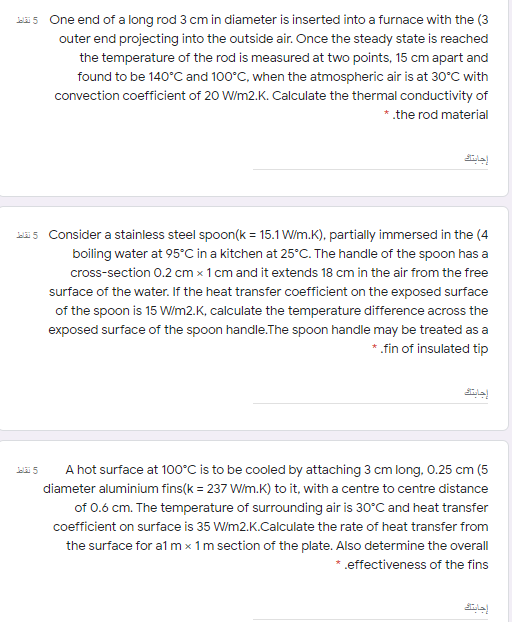255 One end of a long rod 3 cm in diameter is inserted into a furnace with the (3 outer end projecting into the outside air. Once the steady state is reached the temperature of the rod is measured at two points, 15 cm apart and found to be 140°C and 100°C, when the atmospheric air is at 30°C with convection coefficient of 20 W/m2.K. Calculate the thermal conductivity of *.the rod material جابت 265 Consider a stainless steel spoon(k = 15.1 W/m.K), partially immersed in the (4 boiling water at 95°C in a kitchen at 25°C. The handle of the spoon has a cross-section 0.2 cm x 1 cm and it extends 18 cm in the air from the free surface of the water. If the heat transfer coefficient on the exposed surface of the spoon is 15 W/m2.K, calculate the temperature difference across the exposed surface of the spoon handle. The spoon handle may be treated as a * fin of insulated tip جاتے دند A hot surface at 100°C is to be cooled by attaching 3 cm long. 0.25 cm (5 diameter aluminium fins(k = 237 W/m.K) to it, with a centre to centre distance of 0.6 cm. The temperature of surrounding air is 30°C and heat transfer coefficient on surface is 35 W/m2.K.Calculate the rate of heat transfer from the surface for almx 1m section of the plate. Also determine the overall * .effectiveness of the fins إجان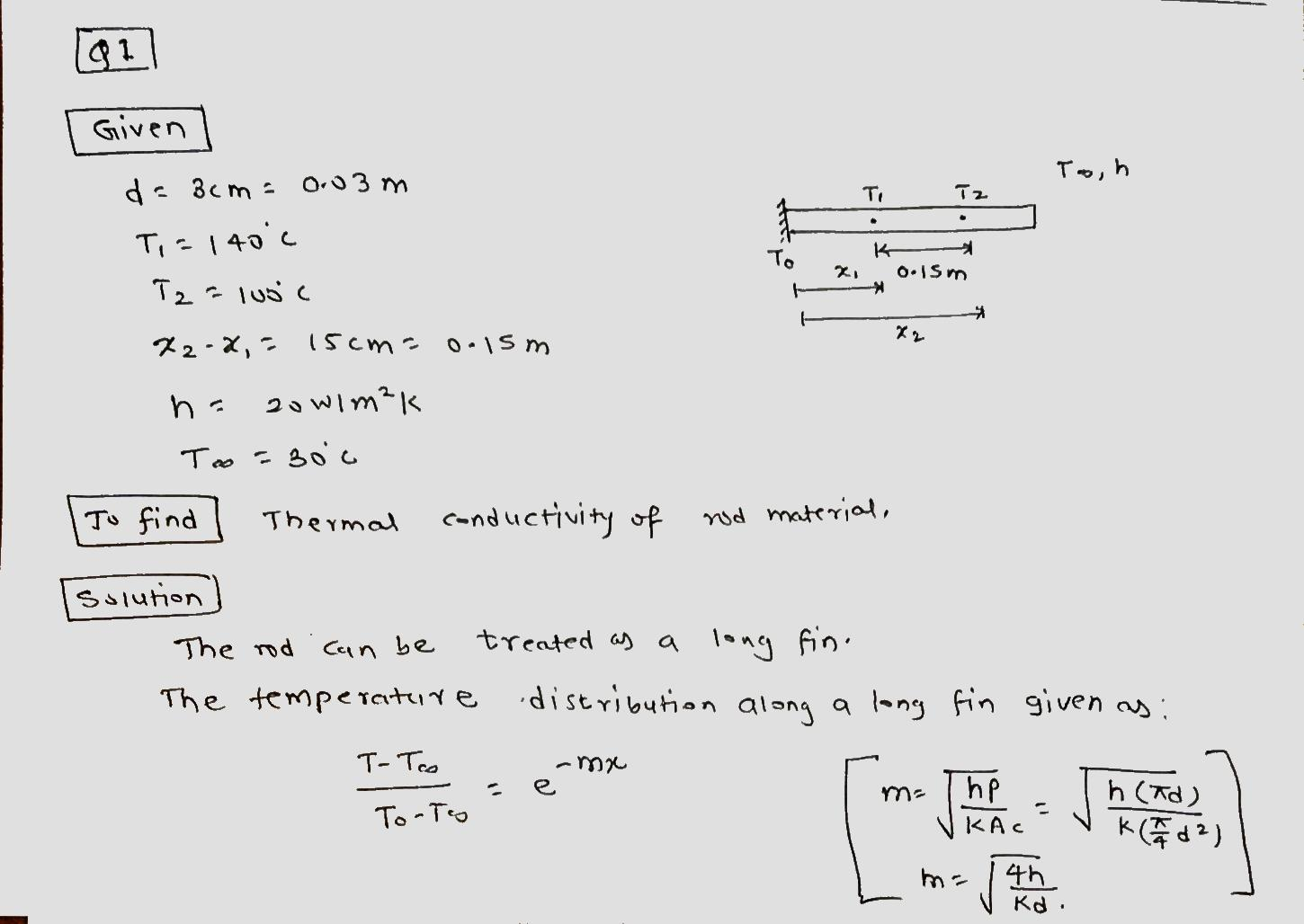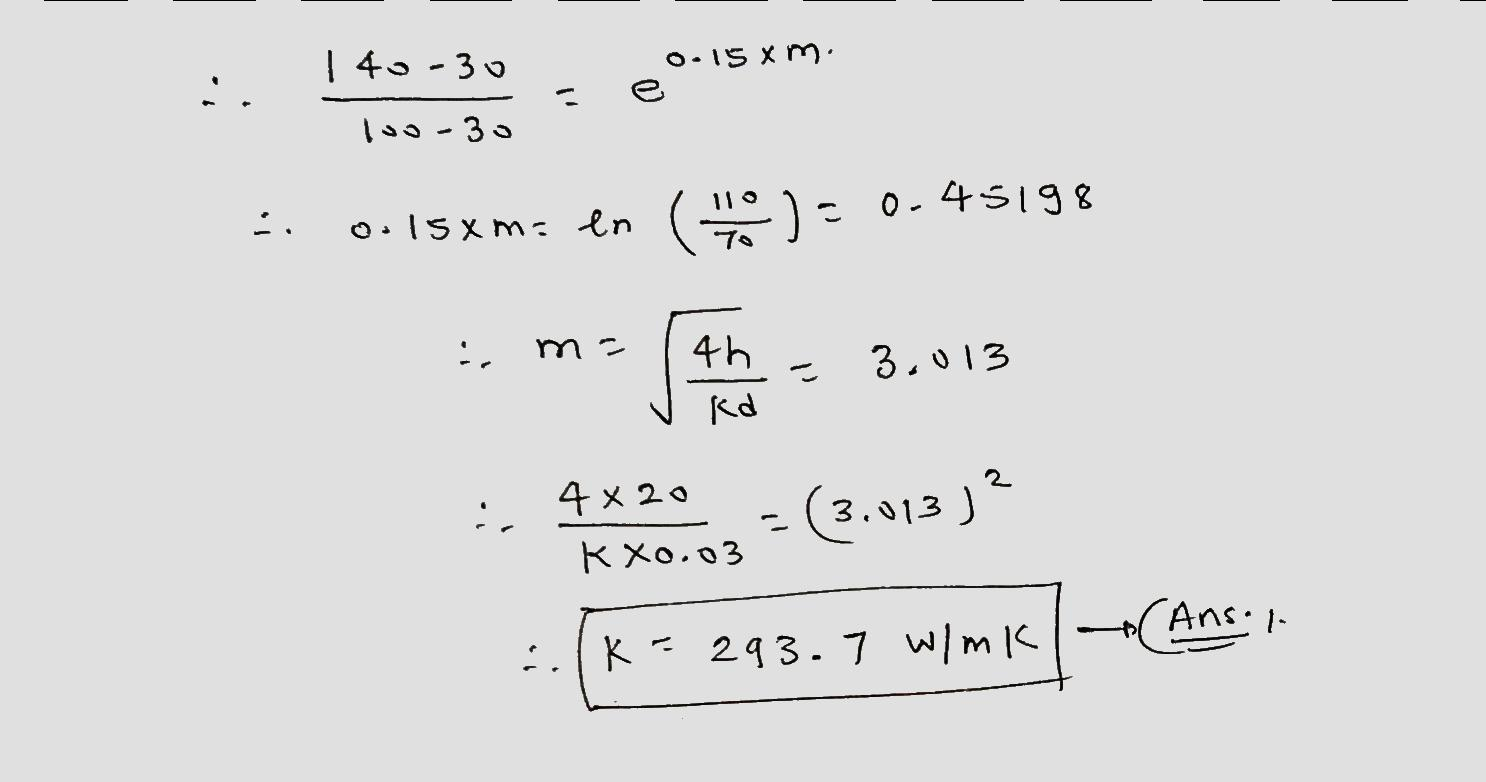##### Add Answer of: 255 One end of a long rod 3 cm in diameter is inserted into a furnace...
Similar Homework Help Questions
• ### Heat transfer bla 5 Consider a stainless steel spoon(k = 15.1 W/m.K), partially immersed in the...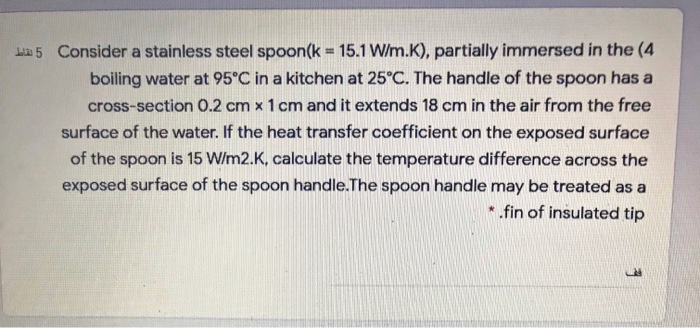Heat transfer bla 5 Consider a stainless steel spoon(k = 15.1 W/m.K), partially immersed in the (4 boiling water at 95°C in a kitchen at 25°C. The handle of the spoon has a cross-section 0.2 cm x 1 cm and it extends 18 cm in the air from the free surface of the water. If the heat transfer coefficient on the exposed surface of the spoon is 15 W/m2.K, calculate the temperature difference across the exposed surface of the spoon...

• ### 2. A hot surface at 120°C is cooled by attaching a 6-cm-long, 0.6-cm-diameter pin fin (k...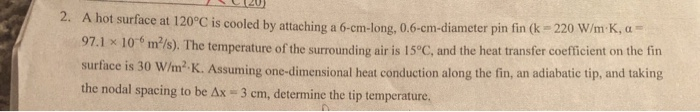2. A hot surface at 120°C is cooled by attaching a 6-cm-long, 0.6-cm-diameter pin fin (k 220 W/m K, a 97.1 x 10 m2/s). The temperature of the surrounding air is 15°C, and the heat transfer coefficient on the fin surface is 30 W/m2 K. Assuming one-dimensional heat conduction along the fin, an adiabatic tip, and taking the nodal spacing to be Ax 3 cm, determine the tip temperature.

• ### A long cylindrical rod of diameter 220 mm with thermal conductivity 0.5 W/m-K expe riences uniform...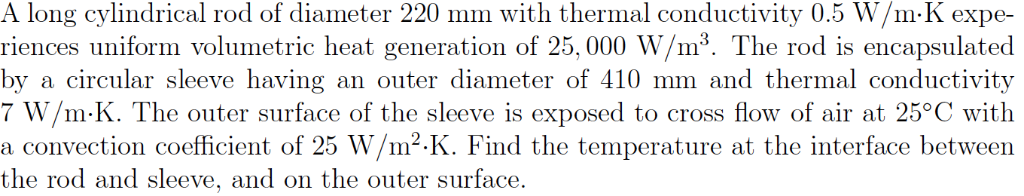A long cylindrical rod of diameter 220 mm with thermal conductivity 0.5 W/m-K expe riences uniform volumetric heat generation of 25,000 W/m3. The rod is encapsulated by a circular sleeve having 7 W/m K. The outer surface of the sleeve is exposed to cross flow of air at 25°C with a convection coefficient of 25 W/m2-K. Find the temperature at the interface between the rod and sleeve, and on the outer surface. an outer diameter of 410 mm and thermal...

• ### A 5-cm-diameter shaft rotates at 4500 rpm in a 15-cm long, 8-cm-outer-diameter cast iron bearing (k...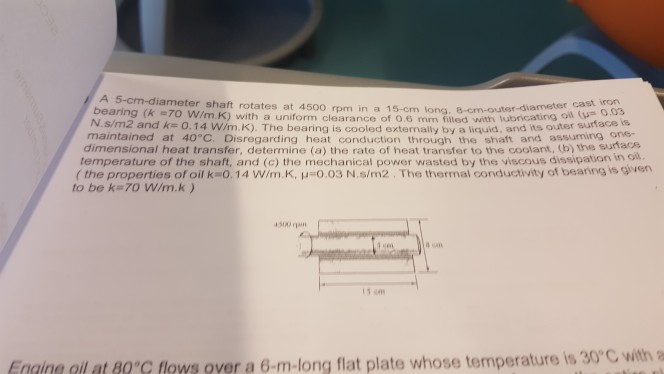A 5-cm-diameter shaft rotates at 4500 rpm in a 15-cm long, 8-cm-outer-diameter cast iron bearing (k =70 W/m.K) with a uniform clearance of 0.6 mm filled wwith tubricating oil (u 0.03 N.s/m2 and k 0.14 W/m.K). The bearing is cooled externally by a liquid, and its outer surface is maintained at 40°C. Disregarding heat conduction through the shaft and assuming one- dimensional heat transfer, determine (a) the rate of heat transfer to the coolant, (b) the sutacs temperature of the...

• ### 5 A hollow sphere of inside radius 30 mm and outside radius 50 mm is (5...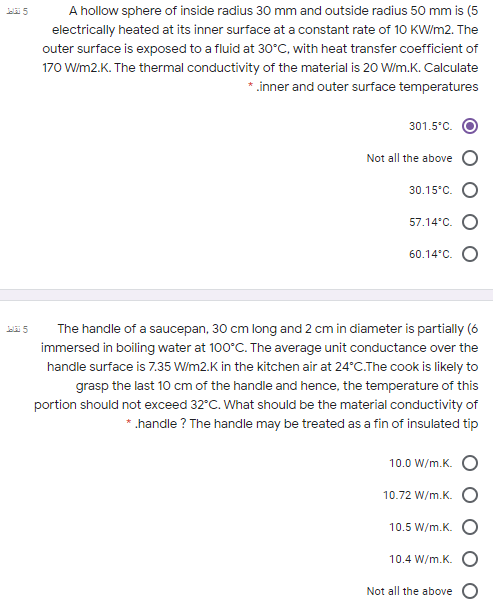5 A hollow sphere of inside radius 30 mm and outside radius 50 mm is (5 electrically heated at its inner surface at a constant rate of 10 KW/m2. The outer surface is exposed to a fluid at 30°C, with heat transfer coefficient of 170 W/m2.K. The thermal conductivity of the material is 20 W/m.K. Calculate *.inner and outer surface temperatures 301.5°C Not all the above 30.15°C. 57.14'c. O 60.14'c. O تقع The handle of a saucepan, 30 cm long...

• ### Consider a stainless steel spoon (k= 15.1 W/m·C) that is partially immersed in boiling water at...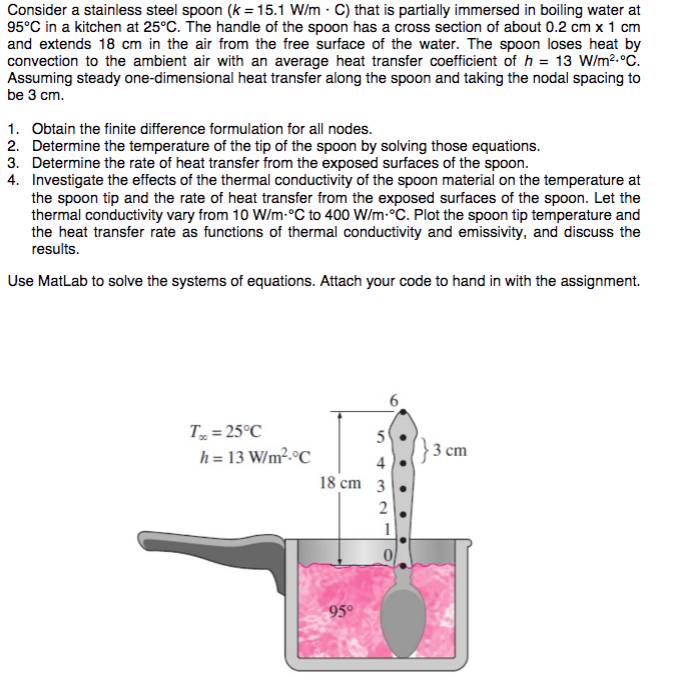Consider a stainless steel spoon (k= 15.1 W/m·C) that is partially immersed in boiling water at 95°C in a kitchen at 25°C. The handle of the spoon has a cross section of about 0.2 cm x 1 cm and extends 18 cm in the air from the free surface of the water. The spoon loses heat by convection to the ambient air with an average heat transfer coefficient of h = 13 W/m2. Assuming steady one-dimensional heat transfer along the...

• ### 265 A person is found dead at 5 p.m. in a room where temperature is 20°C....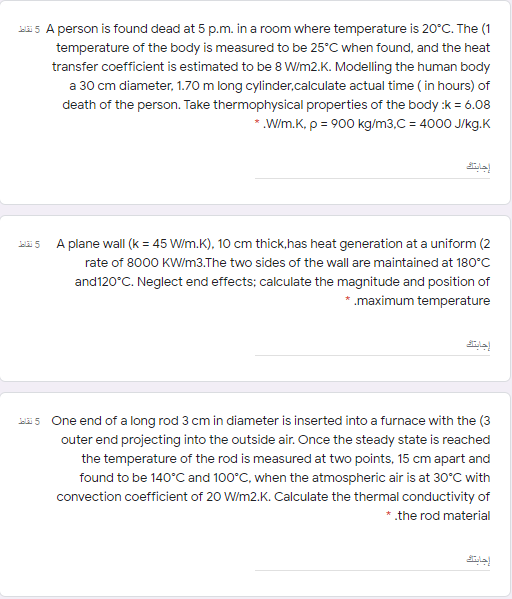265 A person is found dead at 5 p.m. in a room where temperature is 20°C. The (1 temperature of the body is measured to be 25°C when found, and the heat transfer coefficient is estimated to be 8 W/m2.K. Modelling the human body a 30 cm diameter, 1.70 m long cylinder.calculate actual time in hours) of death of the person. Take thermophysical properties of the body :k = 6.08 * W/m.K, p = 900 kg/m3,C = 4000 J/kg.K إجابت...

• ### finite element method 2. Aluminum fins with rectangular profiles (5 mm wide and 1 mm thick) are used to remove heat...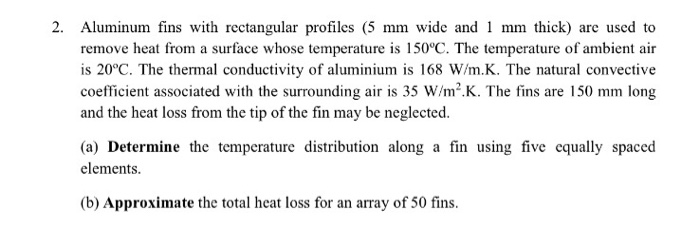finite element method 2. Aluminum fins with rectangular profiles (5 mm wide and 1 mm thick) are used to remove heat from a surface whose temperature is 150°C. The temperature of ambient air is 20°C. The thermal conductivity of aluminium is 168 W/m.K. The natural convective coefficient associated with the surrounding air is 35 W/m2.K. The fins are 150 mm long and the heat loss from the tip of the fin may be neglected. (a) Determine the temperature distribution along...

• ### 1. A steel tube [k 15 W/(m.°C)] of outside diameter 7.6 cm and thickness 1.3 cm...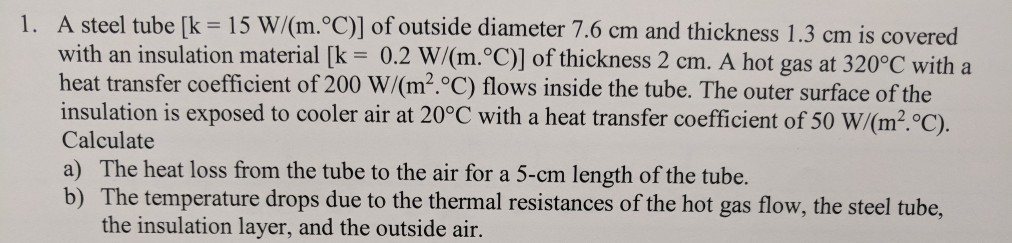1. A steel tube [k 15 W/(m.°C)] of outside diameter 7.6 cm and thickness 1.3 cm is covered with an insulation material [k 0.2 W/(m. C)] of thickness 2 cm. A hot gas at 320°C with a heat transfer coefficient of 200 W/(m2.C) flows inside the tube. The outer surface of the insulation is exposed to cooler air at 20°C with a heat transfer coefficient of 50 W/(m2·°C). Calculate a) The heat loss from the tube to the air for...

• ### Problem 1 (20 marks): A 1-m x 1-m surface at 100 °C is to be cooled...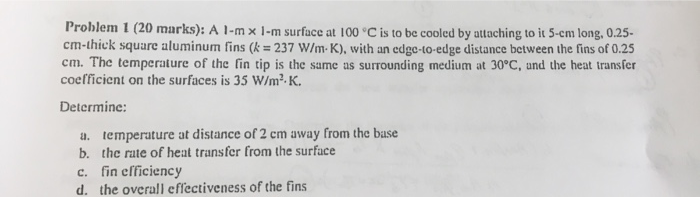Problem 1 (20 marks): A 1-m x 1-m surface at 100 °C is to be cooled by attaching to it 5-cm long, 0.25- cm-thick square aluminum fins (k = 237 W/m- K), with an edge-to-edge distance between the fins of 0.25 cm. The temperature of the fin tip is the same as surrounding medium at 30°C, und the heat transfer coefficient on the surfaces is 35 W/m2 K. Determine: temperature at distance of 2 cm away from the base the...

Free Homework App